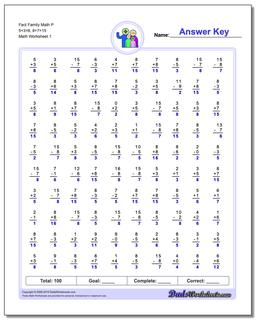# Math Worksheets: Fact Family Math: Fact Family Math: Fact Family Math P 5+3=8, 8+7=15## Fact Family Math P 5+3=8, 8+7=15

PropertyValue
DescriptionFact Family Math P 5+3=8, 8+7=15: These math worksheets have 100 addition and subtraction fact family problems and make for a challenging two minute test.
Resource TypeWorksheet